# Maths# Maths# Maths

## Maths for Psychology

#### Why is Statistics needed in Psychology?

Understanding Statistics is important for several reasons:

• it will enable you to read and interpret research articles for assignments, including interpreting tables, graphs, and statistical analyses.
• it will allow you to conduct your own research including designing quantitative research studies, analysing and interpreting data, and reporting your results in order to advance theory and practice in Psychology.
• Statistical knowledge contributes to the development of your analytical and critical thinking skills. It goes beyond mathematical formulae to understanding underlying relationships between variables in the study of complex human behaviour.

#### What you need to know? What you will be required to do?

• learn statistical concepts and relevant Maths formulae
• interpret results presented through computer software and sometimes use that software for data analysis. The most commonly used software to conduct statistical analysis in Psychology is SPSS
• carry out statistical analysis by hand calculation.

#### For detailed help with Statistics:

If you have not completed Advanced Maths in final years of secondary education, you are encouraged to self-test your Maths foundation skills. Then check you are confident with the concepts covered in this guide. If you identify a concept you need help with, you can find services on the Maths support section and on the Statistics page.

For a thorough preparation for studying STA1STM Statistical Methods as part of your Psychology degree, see the Maths Hub.

### Basic statistical concepts

This section introduces some relevant research concepts that will be covered in your Statistics subjects.

#### Population and sample: sampling methodPsychologists usually study a sample of people in order to make claims about the population as a whole. For example, if a researcher would like to know what type of university student is more likely to experience stress before exams (e.g. males or females, young or mature age students…), a representative group of students would be investigated as it would be impossible to access all university students. The entire group of people about whom we want to make a statement (all university students) is the population; the group of students who actually took part in the research is called the sample.

#### Two branches of statistics: descriptive and inferential

There are two main branches of statistical methods, descriptive statistics and inferential statistics.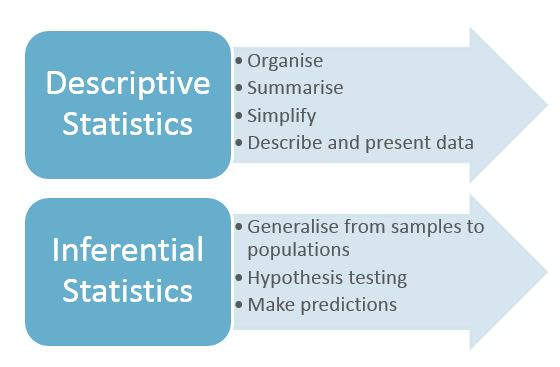Descriptive statistics are used to summarise and describe data. They go beyond averages and frequencies, and also include measures of variability in the data.

For example, if you achieve 20 out of 40 on a test, and the average score on the test was 28/40, you know that you did worse than the average. However, the average does not tell you whether you did much worse than other students, or only slightly worse. This information is given by the variability in the data. For instance, if 95% of students performed between 24 and 34 out of 40, your score of 20 is not so far from the range of scores of other students.

Inferential statistics used to draw conclusions and make inferences about a larger group of individuals (population) based on an investigation of a study sample of that population. Inferential statistics include diverse statistical techniques to assess the reliability of the results obtained in the study sample, and to draw conclusions and generalisations about a population.

For example, to assess whether there are significant differences between groups of university students (e.g. males and females) in their levels of stress before exams, inferential statistics need to be employed.

#### Levels of measurement

Researchers collect different types of data, and these data are measured at different levels. This means that there are different types of measurement scales which will influence the type of statistical analysis to be conducted.

Consider this example of data collected using a questionnaire: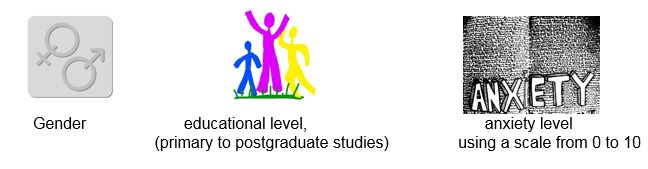In the first and second question, the data collected is non-numerical; that is, the researcher is collecting data about groups (males and females) and levels of education. The main difference between the first and second question is that, in the second question, participants can be ranked according to their educational level.

The third question collects numeric data about the anxiety level of participants, measured using a scale. These questions represent the main types of measurement  of data.

#### Arithmetic and algebra for statistics:

Positive and negative numbers

Numbers Can be Positive (+)  or Negative (-):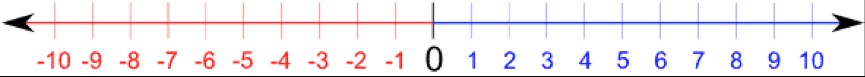In research studies, factors can found have a positive (+) or negative (-) relationship with each other. For example attitudes of long-term friends to certain issues such as abortion are more likely to be similar than to those of a stranger. This illustrates a positive relationship.

What does 'equal' really means?

The equals sign = means that both sides of an equation have the same value. Therefore both sides of an equation must be kept balanced. The balanced scales demonstrate this.If something is added to or subtracted from one side, it should also be added to or subtracted from the other side. If one side is multiplied or divided by that number, the other side must also be multiplied or divided by that number.

#### Basic rules of operations & processes to understand:

[Adapted from Kranzler and Moursund (1995)]

Addition and subtraction of positive and negative numbers

Rule 1: if a digit does not have a + or – sign, it is inferred it is a positive number

Rule 2: When adding up numbers with the same sign (+ or -) put that common sign as a prefix

Rule 3: When adding up numbers with a mixture of signs, add up the +ve numbers and add up the    –ve numbers, then subtract the smaller sum from the larger sum with the sign of the larger sum as the prefix in the answer.

Rule 4: Subtracting a +ve number is the same as adding a –ve number.

Rule 5: Subtracting a –ve number results in a +ve answer.

Multiplication and division of +ve and -ve numbers

Rule 6: Multiplying or dividing two –ves results in a positive answer.

Rule 7: Multiplying or dividing numbers with different signs gives a –ve answer

Rule 8: For a long list of numbers to be multiplied or divided, follow the rules 6  & 7,  and make the calculations in pairs

#### Fractions

Rule 9: A fraction symbolises a division of the number above the line (numerator) being divided by the number below the line (denominator)

Rule 10: Any number can be made a fraction by making a denominator of 1

Rule 11: To multiply fractions, multiply the numerators together and multiply the denominators together

Rule 12: To divide by a fraction, invert the fraction and continue as for Rule 11

Rule 13: To add or subtract a fraction, find the common denominator of both fractions then proceed

Decimals and percentages

Rule 14: a decimal indicates the fraction of 10, 100, 1000 and so on, when those numbers are denominators.                       E.g., .2 =­ 2/10     .02 = 2/100

Rule 15: When a number is less than one, write a 0 before the decimal point 0.2

Rule 16:  Rounding off is needed when converting some fractions to decimals because there are endless digits in the answer. If 1.414 is rounded off to 2 decimal places it becomes 1.41 because if the final digit  is less than (< ) 5 that digit is discarded. However 1.416 rounded off to 2 decimal places becomes 1.42 because if the final digit is greater than (>) 5 the second last digit is increased by 1.

If the final digit is exactly 5, e.g., 1.425 it is discarded then should the second last digit of the number be even number, is kept e.g., 1.42. However if it is odd, e.g., 1.475 it rounded up by 1 e.g., 1.48.

Even:  1.425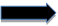1.42         Odd: 1.475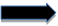1.48

Exponents, powers and roots

An exponent or power is written like this 32. This means 3 is multiplied by itself or squared (2) 3x3 = 9. 33 means 3 is multiplied by itself 3 times or cubed. If the exponent is greater than 3  (35) the number is said to be raised to the power of 5. (See Table 1)

Table 1 Exponents

 3 exponent Expression Expanded form Answer 30 Zero power of 3 1 31 3 to the power of 1 3 3 32 3 squared 3x3 9 33 3 cubed 3x3x3 27 34 3 to the power of 4 3X3X3X3 81

A square root is written like this √9. The square root of a number is the number, that when squared, equals the radicand number inside the √ . This means √3x3 = 3. In other words 9 results from 3 being multiplied by 3. (See Table 2)

Table 2 Roots

 Roots Expression Expanded form Answer √9 Square root of 9 √3x3 3 3√27 Cubed Root of 27 √3X3X3 3 4√81 Fourth Root of 81 √3x3x3x3 3

#### Order of computation- BODMAS rule

Rule 17:  In an equation where there is a long list of processes, order the steps by following this rule Brackets/of/Divide/Multiply/Add/Subtract

Kranzler, G., & Moursund, J. (1995). Statistics for the terrified. Englewood Cliffs, NJ Prentice Hall.

#### Histograms or bar graph

Frequency is shown on the vertical axis. The values of the variables are represented on the horizontal axis. The height of each rectangle (bar) coming up from the horizontal axis is the frequency of occurrence of that value. There is an extra score one number below the bottom score in the range and one number above the top score in the range.Fig 2. Example of histograms. Adapted from Pallant, J. (2013). SPSS survival manual: A step by step guide to data analysis using IBM SPSS (5th ed.). Sydney, Melbourne, Auckland, London: Allen & Unwin

#### Frequency polygon

This is another form of representation of frequency but the bar for each score is replaced with a point directly above each score on the horizontal axis. Then the points for each score are connected in sequence by straight lines. The line starts on the point 1 number below the lowest score and finishes one number above the highest score. These types of graphs can be overlaid to make comparisons between groups of test scores.Fig 3. Example of frequency polygon

#### Normal distribution curve

This is an expression of typical behaviour distribution, called normal probability and is symmetrical with a cluster about the mean. The normal distribution curve represents a smoothed normal histogram and the area between the curve and the horizontal axis represents all of the measurements in any distribution.

68% of all the scores are within +/- 1 of the mean

95% of all scores are within +/- 2 of the mean

99.7% of all the scores are within +/- 3 of the mean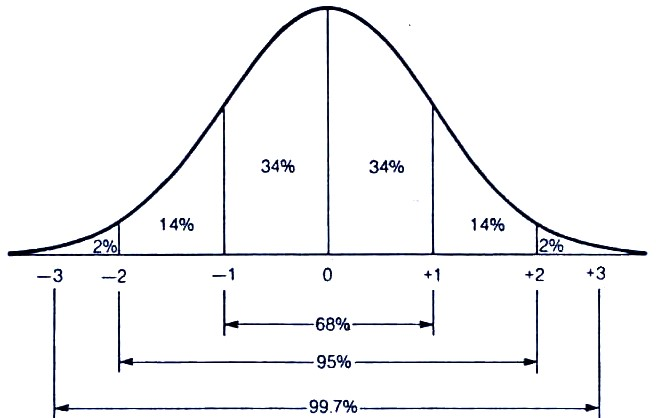Fig 4. Normal distribution curve

#### Kurtosis: Mesokurtic, Leptokurtic or Platykurtic distribution

Scores of smaller groups may not be symmetrical and instead produce a skewed curve.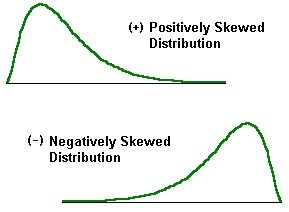Fig 5. Skewed distribution curves

The other results can be a kurtosis where the curve may be more peaked around the mean (Leptokurtic) or flattened (Platykurtic) around the mean. Leptocurtic curves indicate a data set which is clustered around the mean. Mesokurtic curves indicate a normally distributed data set. Platykurtic curves indicate a data set that is highly dispersed.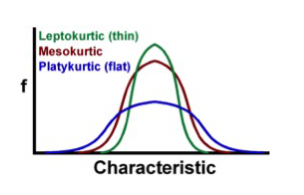Fig 5. Types of distribution curves

#### High positive correlation scatter graph

A scatterplot is a plot of paired (x, y) data with a horizontal x-axis and a vertical y-axis. Each individual pair is plotted as a single point. A scatter plot is used to visualise the relationship between two variables.

This graph implies that a person who scores high in Test X will also score high on Test Y. Note the slope is upward (as it moves to the right) indicating positivity. The more closely the plotted points fall on a straight line cutting the graph diagonally, the greater the correlation.Fig 6. High positive correlation scatter graph. Adapted from Pallant, J. (2013). SPSS survival manual: A step by step guide to data analysis using IBM SPSS (5th ed.). Sydney, Melbourne, Auckland, London: Allen & Unwin

#### Minimal relationship scatter graph

There is no clear relationship between high and low, high and high and low and low scores in this graph.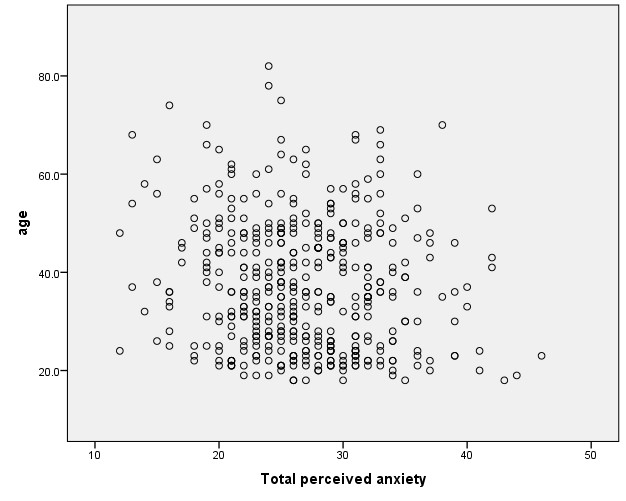Fig 7. Minimal relationship scatter graph. Adapted from Pallant, J. (2013). SPSS survival manual: A step by step guide to data analysis using IBM SPSS (5th ed.). Sydney, Melbourne, Auckland, London: Allen & Unwin

#### High negative correlation scatter graph

This graph shows that a person who scores high in Test X will probably score low in Test Y. Similarly, those that score high in Test Y will score low in Test X. The slope of this graph is downwards (as it moves to the right) and indicates negativity. The more scattered the points are, the lower the correlation.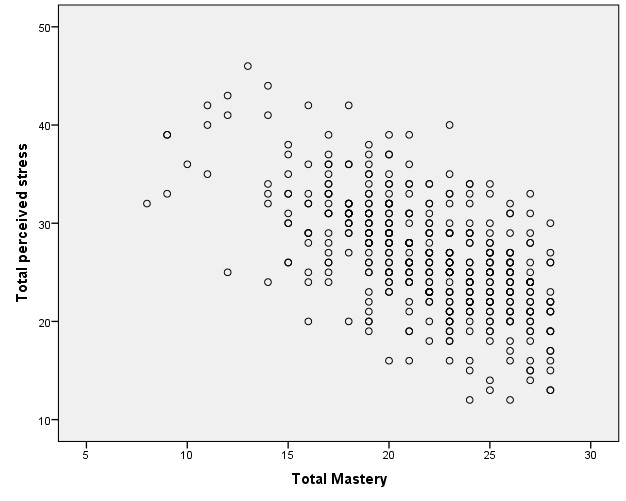Fig 8. High negative correlation scatter graph. Adapted from Pallant, J. (2013). SPSS survival manual: A step by step guide to data analysis using IBM SPSS (5th ed.). Sydney, Melbourne, Auckland, London: Allen & Unwin

#### Statistical Measurements used in Foundation Psychology

Foundation subjects regularly use statistical measures such as: mean that summarises raw data that has been collected and organised when testing a hypothesis; standard deviation that shows variability from the mean; correlation coefficients that help measure possible relationships between variables that may be influencing the test results; risk ratios that measure the level of chance of something occurring or not; and the statistical significance testing or probability of the observed result being due to chance shown with p values.  These measurements may be demonstrated in Tables or Graphs.

Mean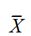is when all scores that have been collected are added together, then the total ∑x is divided by the number of scores n when x = a test score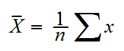Standard deviation  is a measure of variability between groups that is comparable to the original measures obtained by using this formula

s = √s2  when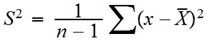Correlation coefficients are the numbers that indicate degree of relatedness between 2 or more variables.

0 means there is no correlation between the variables

-1 or +1 means maximum relationship between variables

-1 indicates a negative relationship

+1 indicates a positive relationship

Pearson product–moment r ) is an example of a correlation coefficient. r is a measure of the relationship between 2 variables where when r=1.00 means perfect correlation (but not necessary the cause) and where r= 0 there is no correlation.

Coefficient of Determination  is calculated using r and is the square of the correlation co-efficient. The result can be converted to % to explain to what extent the variability of one factor (eg IQ) is accounted for by the variability of another factor (eg Grade Point Average).

Cohen’s Rule of Thumb, Cohen’s d expresses the absolute change relative to the standard deviation. Calculate it by taking the absolute difference (mean difference between experimental and control group) and divide it by the standard deviation. This gives the SMD or Standardised mean difference or effect size.

SMD of 0.02 or less represents a small change

SMD of 0.50 represents a moderate change

SMD of 0.80 represents a large change

t-test  is an inferential statistic used to determine whether the means of two groups of scores differ to a statistically significant degree. It is used to test the null hypothesis, or that there is no difference in the means of the two groups. The t-test is mainly applied to independent samples where subjects are assignment randomly to one group or another. A measurement of .05 or 5% is large enough to be statistically significant which means the null hypothesis can be rejected. This is written as p<.05.  One–tailed t-test/directional test is used when the direction of the difference is predicted before the data is collected. Two-tailed t-tests are used when there is some doubt about the results being significant prior to testing.

Risk ratios  refer to the risk of an event occurring such as the odds of developing cancer from smoking

Values greater than 1.0 indicate increased risk.

Values less than 1.0 indicate reduced risk.

Values equal to 1.0 indicate the risk is no better than chance.

P values   Research scientists generally set the significance value for their experiments at 0.05, or 5 percent. This means that experimental results that meet this significance level have, at most, a 5% chance of being the result of pure chance. In other words, there's a 95% chance that the results were caused by the scientist's manipulation of experimental variables, rather than by chance. For most experiments, being 95% sure about a correlation between two variables is seen as "successfully" showing a correlation between the two. Put simply:

if the p<.05 , the differences in results between cohorts are statistically significant and unlikely to have occurred by chance, but if the p>.05 any difference is due to chance.

Ƒ – frequency

Raw data can be organised to show frequency of particular scores. The results can be arranged in a frequency distribution table or a graph. This shows how many times a particular score occurs.

Table 1  Frequency with raw data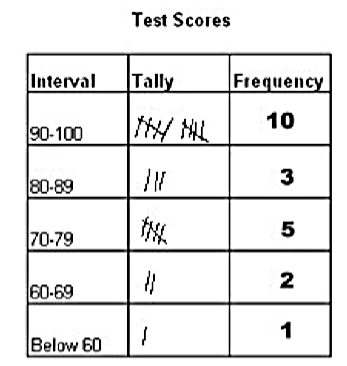Table 2 Frequency without raw dataTable 3 Frequency with NX – any value of the variable under consideration.

In the example this would be any test score

#### Help with statistics skills for Psychology

If you have not completed Advanced Maths in final years of secondary education, you are encouraged to self-test your Maths foundation skills. Then check you are confident with the concepts covered in this guide. If you identify a concept you need help with, you can find services on the Maths support section.

For more detailed explanations of concepts try the Statistics Page.

For a thorough preparation for studying STA1STM Statistical Methods as part of your Psychology degree, find out more about SHE Maths Skills Program, Maths Skills for Statistics module.